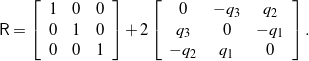# Infinitesimal rotations

An infinitesimal rotation is defined as a rotation about an axis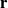through an angle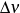that is very small: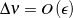, where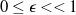. Rotations of this type play a role in defining stiffness matrices (see  and references therein) and in numerical schemes that feature incremental updates to rotations and angular velocities (e.g., see ). Infinitesimal rotations differ from their finite counterparts in the simplicity of their representation and in the fact that the composition of infinitesimal rotations is commutative.

Contents

## Euler’s representation

From the assumptions on the smallness of the angle of rotation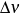, it follows that

(1)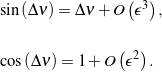There is no corresponding smallness restriction on the axis of rotation. With the help of (1), we find that if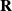is the rotation tensor for an infinitesimal rotation, then Euler’s representation simplifies to

(2)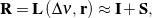where the skew-symmetric tensor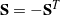is obtained from the rotation vector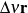:

(3)That is, the axial vector ofis. The representation (2) is also easily established from the exponential map representation of a rotation tensor. It is also interesting to note that the inverse of an infinitesimal rotation has the simple representation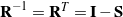. In matrix form, the infinitesimal rotation (2) has the representation

(4)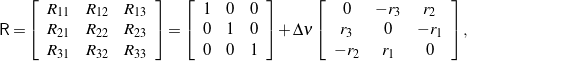where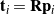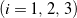and

(5)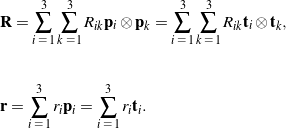To first order in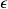, it can be shown from (2) that. Consequently, for infinitesimal rotations, the distinction between these two sets of basis vectors is typically ignored, a feature which is useful when considering the composition of infinitesimal rotations.

## The Euler angle representation

From either of the representations (2) or (4), it follows in a straightforward manner that the composition of two infinitesimal rotations is commutative. As a result, suppose we have three infinitesimal rotations about three axes:

(6)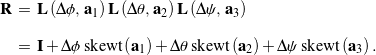Assuming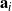are mutually orthogonal (i.e.,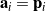), we see from (4) that the matrix representation for the resulting compound rotation is

(7)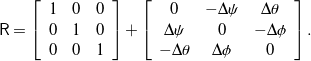We can use either (6) or (7) to infer the representation of an infinitesimal rotation for any one of the 12 sets of Euler angles. For example, (7) is the matrix representation for a infinitesimal rotation parameterized by a set of 3-2-1 Euler angles.

## The Euler-Rodrigues and quaternion parameterizations

If a set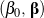of Euler-Rodrigues symmetric parameters or, equivalently, a unit quaternion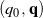is used to parameterize an infinitesimal rotation, then we deduce from the developments in the section on the Euler-Rodrigues and quaternion parameterizations that

(8)The resulting representation of the rotation tensoris a linear function of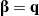:

(9)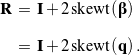The matrix representation of this expression is easily inferred from (4). For example, in terms of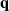,

(10)